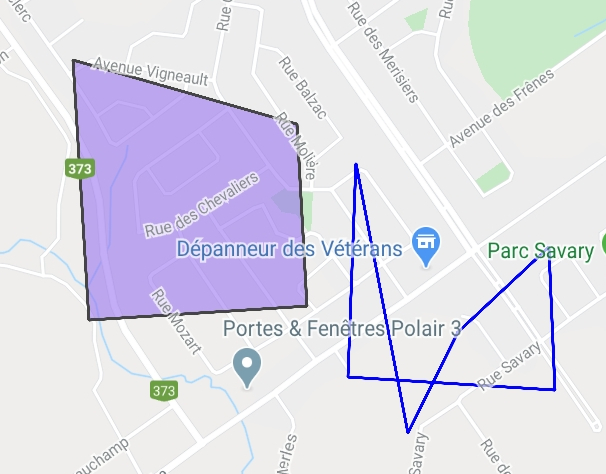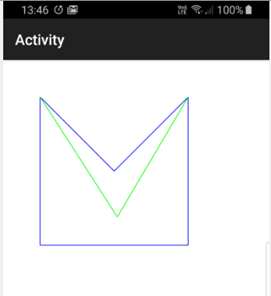Android Question[SOLVED] GoogleMapsExtra polygon cross line

yfleury

Active MemberHere 2 polygon. The right one, border cross another one.
How to detect this programaticaly?

Staff member

oparra

Well-Known Member
I only see a polygon.
that their lines cross
or not ?

I see 3 segments of lines crossing

Erel post is fine

Last edited:

Other:

yfleury

Active Member
I take a look on all link. But it is too complicate for my knowledge to translate to b4a

oparra

Well-Known Member
are classes of languages (calculation of geometry) and you can execute, edit and copy.
and Translate to B4Xyfleury

Active Member
Thanks @oparra But I am unable to translate that. I unknow how to do that. My java skill is 0/10

oparra

Well-Known Member
Tell me what are you really looking for?

And I'll see if we already have it in our classes in B4X.

regards

Last edited:

oparra

Well-Known Member
Indicates the points of the polygon (GoogleMapsExtra)

Latitude and longitude that make up the polygon.

The start point is equal to the end point of the polygon.

regards

oparra

Well-Known Member
Do you want to know the points of the segments (line) that intersect?

yfleury

Active Member
I Just want a boolean if a segment cross another segment. If it true, I show a warning to user "Your polygon is bad because a segment is crossing another segment. Remake the polygon. "

Brian Dean

Active Member
How are the vertices of your polygon stored? If they are held as a list of objects what are the objects?

yfleury

Active Member
I have 2 lists, a list of latitude and a list of longitude

Brian Dean

Active Member
Here is a possible solution. The following code will determine if two lines (eg edges in a polygon) intersect ...

B4X:
' TRUE if line objects L1 and L2 self-intersect
Sub intersects(L1 As line, L2 As line) As Boolean
Return (pointside(L1, L2.p0) <> (pointside(L1, L2.p1))) And (pointside(L2, L1.p0) <> (pointside(L2, L1.p1)))
End Sub

' Determine on which side of line L point P lies
Sub pointside(L As line, P As point) As Int
Dim a As Float
a = ((L.p1.x - L.p0.x) * (P.y - L.p0.y)) - ((P.x - L.p0.x) * (L.p1.y - L.p0.y))
If (a > 0) Then Return 1
If (a < 0) Then Return -1
Return 0
End Sub

The code uses Line and Point objects as defined in the next code segment. You will have to convert your lists of latitude and longitude values into lines. Start by combining the longitude-latitude pairs into x-y points, then convert the x-y points into lines ...

B4X:
Type point (x As Float, y As Float)
Type line (p0 As point, p1 As point)

. . . . .
. . . . .

' Convert a list of point objects to a list of line objects
Sub makeLines(points As List) As List
Dim result As List
result.Initialize
Dim p, oldP As point
Dim first As Boolean = True
For Each p As point In points
If Not(first) Then
End If
oldP = p
first = False
Next
Return result
End Sub

Public Sub createLine (p0 As point, p1 As point) As line
Dim L As line
L.Initialize
L.p0 = p0
L.p1 = p1
Return L
End Sub

Finally you have to test each line in the polygon against the other lines to determine if any intersect. Note that if the polygon has less than four points then no sides can intersect, and you must not test adjacent sides. The [validShape] routine below expects a list of point objects and builds these into a list of line objects.

B4X:
' Return TRUE if the closed path represented by the list of point objects in [shape] do not self-intersect.
Sub validShape(shape As List) As Boolean
If (shape.Size > 4) Then
Dim result As Boolean = True
Dim lines As List = makeLines(shape)
Dim A, B As line
Dim i, j As Int
i = 0
Do While result And (i < lines.Size - 2)
A = lines.Get(i)
For j = i + 2 To lines.Size - 2
B = lines.Get(j)
result = Not(intersects(A, B))
Next
i = i + 1
Loop
Return result
Else
Return True
End If
End Sub

I have had to adapt this code from code that I have used before, so I have not tested it thoroughly. Let me know if you run into any problems. Just a note; if you are going to work with polygons that have very, very many sides then there are better algorithms that this one.

Last edited:
•oparra

klaus

Expert
Or try this one, in the attached test project.Attachments

• TestPolygon.zip
9.8 KB · Views: 89
Last edited:
•yfleury, Erel and oparra

Brian Dean

Active Member
@yfleury - As you are working with Lat/Lon values I suggest that you use Double variables for the point coordinates rather than Float, especially if you are working at metre precision rather than kilometres.

yfleury

Active Member
@yfleury - As you are working with Lat/Lon values I suggest that you use Double variables for the point coordinates rather than Float, especially if you are working at metre precision rather than kilometres.
For segment A I have 2 points, each point have a Lat and lng (each is Double) I don't use LatLng variable.

Segment A Start at (lat1, lng1) stop at (lat2, lng2)
Segment B .....

Brian Dean

Active Member
Don't worry - if you use my code just make this change ....

B4X:
' Replace this line ...
' Type point (x As Float, y As Float)
' with this line ...
Type point(x as Double, y as Double)

Sorry - I should have made that clear. Good luck.

•yfleury

yfleury

Active Member
@Brian Dean I don't test your code. But thanks again. I bookmark it for later.

I test code from code from @klaus It work well and it is simple (only one sub) to implement it to my app.

Thanks to all

Replies
2
Views
228
Replies
1
Views
220